Courses

# Test: Reaction Mechanism- 4

## 30 Questions MCQ Test Organic Chemistry | Test: Reaction Mechanism- 4

Description
This mock test of Test: Reaction Mechanism- 4 for Chemistry helps you for every Chemistry entrance exam. This contains 30 Multiple Choice Questions for Chemistry Test: Reaction Mechanism- 4 (mcq) to study with solutions a complete question bank. The solved questions answers in this Test: Reaction Mechanism- 4 quiz give you a good mix of easy questions and tough questions. Chemistry students definitely take this Test: Reaction Mechanism- 4 exercise for a better result in the exam. You can find other Test: Reaction Mechanism- 4 extra questions, long questions & short questions for Chemistry on EduRev as well by searching above.
QUESTION: 1

### For the reaction below if the concentration of KCN is increased four times, the rate of the reaction will be: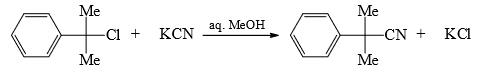Solution:

This is an SN1 reaction, so, rate of the reaction only depends on substrate, it doesn't depend on nucleophile. Hence change in concentration of the nucleophile won't affect the rate.

C is correct.

QUESTION: 2

### The major product formed in the reaction of 1, 3-butadiene with bromine is at high temperature:

Solution:

When 1,3-butadiene reacts with Br2 at high temperature (heating) then 1,4- addition to diene takes place, so mainly 1,4-dibromobut-2-ene is formed. But at low-temperature 1,2-addition dominates and 3,4-dibromobut-1-ene is major product

QUESTION: 3

### The products formed in the following reaction is: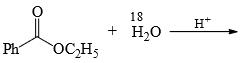Solution:
QUESTION: 4

In the reaction sequence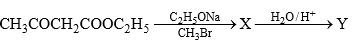The product (Y) is:

Solution:
QUESTION: 5

The major product of the reaction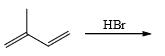Solution:
QUESTION: 6

The major product formed in the following reaction.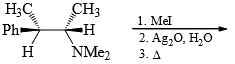Solution:
QUESTION: 7

The major product formed in the following reaction.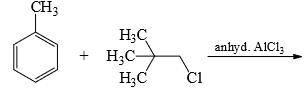Solution:
QUESTION: 8

The major product obtained in the following reaction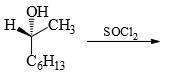Solution:
QUESTION: 9

The major product formed in the following reaction is: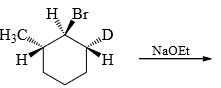Solution:
QUESTION: 10

Choose the correct order of reactivity for dehydration of the given alcohols using concentrated sulfuric acid.

Solution:
QUESTION: 11

Electrophilic nitrations of the following compounds follow the trend: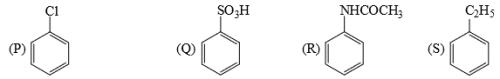Solution:
QUESTION: 12

Elimination reactions of compounds 1 and 2 with sodium ethoxide give two alkenes, A and B, but with different selectivities. Which of the following statements best indicates the most probable outcome?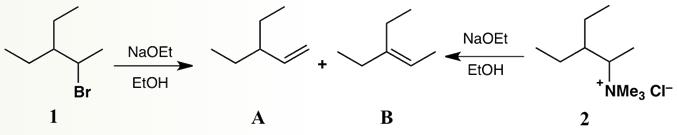Solution:
QUESTION: 13

What would be the final major product of the following chemical reaction if it is carried out twice, one at 5 Co and the second time at 45 C0?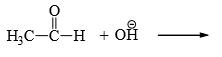Solution:
QUESTION: 14

What is the major product if HBr (in excess) is added to H2C = CH – CH2 – OH

Solution:
QUESTION: 15

The reaction of sodium ethoxide with ethyl iodide to form diethyl ether is termed

Solution:
QUESTION: 16

What is the final product after the following reaction has gone to completion?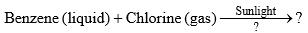Solution:
QUESTION: 17

The major product formed in the following reaction is: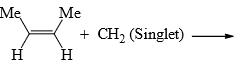Solution:
QUESTION: 18

Among the bromides I-III given below, the order of their reactivity in the SN1 reaction is: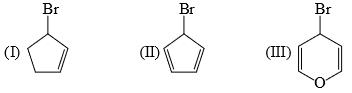Solution:
QUESTION: 19

The major product formed in the reaction benzoic acid with isobutylene in the presence of a catalytic amount of sulfuric acid is:

Solution:
QUESTION: 20

Among the following compounds, the one that undergoes deprotonation most readily in the presence of a base, to form a carbanion is:

Solution:
QUESTION: 21

Regarding the saponification of M and N shown below, the correct statement is that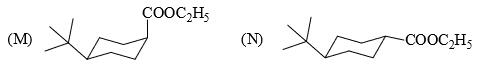Solution:
QUESTION: 22

The major product P formed in the given: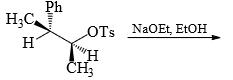Solution:
QUESTION: 23

Iodo-lactonization of β, γ- unsaturated carboxylic acid X with I2 and NaHCO3 gives: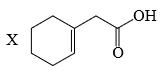Solution:
QUESTION: 24

The major product formed in the following reaction is: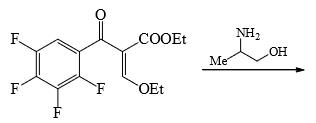Solution:
QUESTION: 25

Predict the major product P in the following reaction: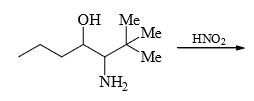Solution:
QUESTION: 26

The major product formed in the following reaction is: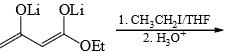Solution:
QUESTION: 27

The major product obtained treatment of compound X with H2SO4 at 80°C is: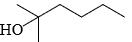Solution:
QUESTION: 28

In the following compound, the hydroxy group that is most readily Methylated with C2H2N2 is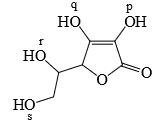Solution:
QUESTION: 29

In the reaction, the major product X is: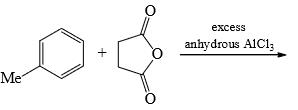Solution:
QUESTION: 30

In the reaction, the major product X is: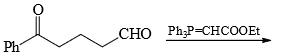Solution: